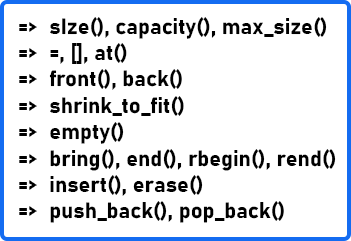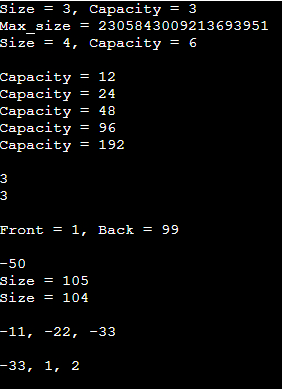# Vector in C++

## Vector in C++ with Examples:

In this article, I am going to discuss Vector and Vector Functions in C++ with Examples. Please read our previous article, where we discussed Iterator Invalidation in C++ Examples.

##### Vector in C++:

Containers are an important part of STL which provide the space where we store the actual data. In the upcoming articles, we will look at different containers provided in C++ STL. Let us begin with a vector

Vectors store the elements contiguously. If you know the position of one of the elements, you can access the elements to the left and right of that. The storage of vectors is handled automatically. For example, initially, you may insert four elements and later you push back a few elements or insert a few elements in the end or in the middle and it goes beyond the capacity then it will automatically reallocate a new space and copy everything and the new elements. So, it is handled automatically.

And it can be expanded or contracted as needed. Vectors occupy more space than static arrays. Since every time new memory allocation does not happen, let’s say you have just one element so it has a capacity of one.Next, you insert two elements and now it does not have space for that so it will allocate a space of 2.Next time when you insert let us say you want to insert 3 then there is no space here so it will let us say allocate a space of 4 beforehand. It is one of the strategies to implement dynamically sized arrays where the strategy is that when the space is full allocate an array of double the size and then copy the elements. So, 1 and 2 will be copied. The third element which you inserted will be pushed here and it still has space of one more elementSo next time you insert 4 no reallocation takes place.But next time you insert 5 it will most likely allocate a space of 8 and copy the fifth element there and it has a space for the remaining three.These all are done for you automatically. You don’t need to manage this. Now let’s look at some of the important functions in vectors.

##### Vector Functions in C++:Size function is present in all the STL containers. It gives you the number of elements that you have inserted but not the actual capacity. The difference between size and capacity is,You can see there is a space of 4 elements but we have inserted only 3 elements. So it has a capacity of 4 and the size is 3. Capacity is the total number of elements that can be inserted in any container. Max_size is the theoretical limit on the maximum number of elements that can be inserted and it will be typically a very large number.

An equal operator (‘=’) can allocate a new vector or initialize a list to a vector. A square bracket (‘[]’) is used to access a vector element using the index. Let us say we want to access the 2nd element of a vector so we will write v  (v is the vector name). Here bound check is not there so even if it has 3 elements and we write v that is the 4th element so it will go ahead and in most scenarios, it may give you zero. So, it will try to access this address and give you some value. At() is also used for the same purpose but here bound check is there so if you try to write v.at(3) then it will give an index out of bound exception.

Front function will return the first element and the back function will return the last element of the vector.

Shrink_to_fit function is used to return the remaining space. So, in this case, we have 3 elements but it has a capacity of 4 elements and we know that we do not need to insert any further. So, what we can do? We can do v.shrink_to_fit() so it will return this memory back and Its capacity will reduce to its size. This can be a very handy function in some scenarios.

Just like size all the containers provide empty function. So you can either write v.size() == 0 to check whether it is empty or not or you can directly call the empty function v.empty(). It returns a Boolean value i.e. true or false.

We have already seen begin, end, rbegin, and rend in our earlier article where we saw iterator functions. So, begin returns an iterator that is pointing to the first element of the vector and returns an iterator to an imaginary slot after the last element of the iterator of the vector. rbegin is a reverse order traversal. It will return a reverse iterator which is pointing to the last element and rend is an iterator that will be pointing to a space before the first element.

Then we have insert and erase functions. If we want to insert at a particular position let’s say we want to insert at the third position so we will write v.insert(v.begin() + 2). Here we have to provide the iterator. There are multiple versions of this either you provide a value or you provide two more iterators. So, you read from some other container, and from that container let’s say you want to insert everything from the 2nd to 5th index so it will provide these two iterators of that container. It1 and it2 so both of these are valid as either a value or two iterators. The first iterator is the position where you have to start inserting similarly for erasing you need to provide one iterator or you can provide a range of iterators so in this case [it1, it2). ‘[‘ is open interval ‘)’ is a closed interval. So it1 will be included and it2 will not be included. A similar is applicable for erase function.

Push_back inserts in the end and pop_back removes one element from the end. Let us see the example of all of these functions.

##### Example to Understand Vector Functions in C++:
```#include <iostream>
#include <vector>
#include <list>
using namespace std;

int main()
{
vector<int> v = {1,2,3};
// size() and capacity()
cout << "Size = " << v.size() << ", Capacity = " << v.capacity() << endl;
// max_size()
cout << "Max_size = " << v.max_size() << endl;

v.push_back(4);
cout << "Size = " << v.size() << ", Capacity = " << v.capacity() << endl;

cout << endl;
int cap = v.capacity();
for(int i = 0; i < 100; i++){
v.push_back(i);
if(cap != v.capacity()){
cap = v.capacity();
cout << "Capacity = " << cap << endl;
}
}

// [] and at
cout << endl;
cout << v << endl; // no bound check
cout << v.at(2) << endl; // it will check bound check

// front() and back()
cout << endl;
cout << "Front = " << v.front() << ", Back = " << v.back() << endl;

// insert() and erase()
cout << endl;

v.insert(v.begin() + 3, -50);
cout << v << endl;
cout << "Size = " << v.size() << endl;
v.pop_back();
cout << "Size = " << v.size() << endl;

cout << endl;
list<int> ll = {-11, -22, -33};
v.insert(v.begin(), ll.begin(), ll.end());
cout << v << ", " << v << ", " << v << endl;

cout << endl;
v.erase(v.begin(), v.begin() + 2); // it will not include v.begin() but include v.begin() + 2
cout << v << ", " << v << ", " << v << endl;

return 0;
}
```
###### Output: